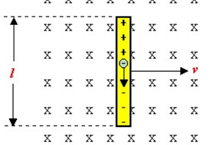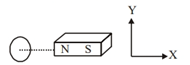Physics
Easy

Question

# The split rings are also known as ____.

## BrushesArmatureCommutatorMagnetsHint:

## The correct answer is: Commutator

Physics

### The device which converts mechanical energy into electrical energy is called a/an _____.

1. Electric motor
2. Electric generator
3. Transformer
4. Inverter

### The device which converts mechanical energy into electrical energy is called a/an _____.

1. Electric motor
2. Electric generator
3. Transformer
4. Inverter
Physics

### When the plane of the armature of an AC generator is parallel to the field, in which is it rotating?

1. Flux linked is maximum while induced emf is zero
2. The flux linked with it is zero, while induced emf is maximum
3. Both the flux linked and induced emf in the coil are zero.
4. Both the flux and emf have their respective maximum values

### When the plane of the armature of an AC generator is parallel to the field, in which is it rotating?

1. Flux linked is maximum while induced emf is zero
2. The flux linked with it is zero, while induced emf is maximum
3. Both the flux linked and induced emf in the coil are zero.
4. Both the flux and emf have their respective maximum values
Physics

### Electric generators can produce

1. Only AC current
2. Only DC current
3. Both AC and DC current
4. No current

### Electric generators can produce

1. Only AC current
2. Only DC current
3. Both AC and DC current
4. No currentPhysics

### The essential difference between an A.C. generator and a D.C. generator is that

1. A.C. generator has an electromagnet, while a D.C. generator has a permanent magnet.
2. D.C. generator will generate a higher voltage
3. A.C. generator will generate a higher voltage
4. A.C. generator has slip rings while the D.C. generator has split rings.

### The essential difference between an A.C. generator and a D.C. generator is that

1. A.C. generator has an electromagnet, while a D.C. generator has a permanent magnet.
2. D.C. generator will generate a higher voltage
3. A.C. generator will generate a higher voltage
4. A.C. generator has slip rings while the D.C. generator has split rings.
Physics

### Choose the correct option by reading the following statements: Assertion: The induced emf in a conducting loop of wire will be nonzero when it rotates in a uniform magnetic field. Reason: The emf is induced due to a change in magnetic flux.

1. Both assertion and reason are true, but the reason is not the correct explanation of the assertion.
2. The assertion and reason both are false.
3. Both assertion and reason are true, and the reason is the correct explanation of the assertion.
4. Assertion is false but the reason is true.

### Choose the correct option by reading the following statements: Assertion: The induced emf in a conducting loop of wire will be nonzero when it rotates in a uniform magnetic field. Reason: The emf is induced due to a change in magnetic flux.

1. Both assertion and reason are true, but the reason is not the correct explanation of the assertion.
2. The assertion and reason both are false.
3. Both assertion and reason are true, and the reason is the correct explanation of the assertion.
4. Assertion is false but the reason is true.
Physics

### An induced emf is induced in the circuit whenever the magnetic flux is related to a coil change:I. for short, the e.m.f. lastsII. for an extended period of timeIII. for as long as the flux is changingThe correct statement(s) is/are

1. II and III
2. III only
3. I and III
4. I and II

### An induced emf is induced in the circuit whenever the magnetic flux is related to a coil change:I. for short, the e.m.f. lastsII. for an extended period of timeIII. for as long as the flux is changingThe correct statement(s) is/are

1. II and III
2. III only
3. I and III
4. I and IIPhysics

### Read the following statements and choose the correct option.Assertion: The figure shows:a metallic conductor moving in a magnetic field. The induced emf across its ends is non zero.Reason: The induced emf across the ends of a conductor is given by Blv sinθ.

1. Assertion is correct, the reason is correct; the reason is not a correct explanation for assertion
2. The assertion is correct, the reason is incorrect
3. Assertion is correct, the reason is correct; the reason is a correct explanation for assertion
4. Assertion is incorrect, reason is correct
Physics

### Choose the correct option from the following statements.Assertion: The movement of a conductor in a magnetic field can induce an emf.Reason: A change of the magnetic field might cause an emf.

1. Assertion is incorrect, reason is correct.
2. The assertion is correct, the reason is correct; the reason is not a correct explanation for assertion.
3. The assertion is correct, the reason is incorrect.
4. The assertion is correct, the reason is correct; the reason is a correct explanation for assertion.

### Choose the correct option from the following statements.Assertion: The movement of a conductor in a magnetic field can induce an emf.Reason: A change of the magnetic field might cause an emf.

1. Assertion is incorrect, reason is correct.
2. The assertion is correct, the reason is correct; the reason is not a correct explanation for assertion.
3. The assertion is correct, the reason is incorrect.
4. The assertion is correct, the reason is correct; the reason is a correct explanation for assertion.
Physics

### Consider a coil and a magnet.Current is induced in the coil when:I. coil and magnet both are at rest.II. the coil is at rest and the magnet moves along x.III. magnet is at rest and coil moves along x.IV. both coil and magnet move along y with the same speed.The correct statements are:

1. III and IV
2. I and IV
3. I and II
4. II and III

### Consider a coil and a magnet.Current is induced in the coil when:I. coil and magnet both are at rest.II. the coil is at rest and the magnet moves along x.III. magnet is at rest and coil moves along x.IV. both coil and magnet move along y with the same speed.The correct statements are:

1. III and IV
2. I and IV
3. I and II
4. II and IIIPhysics

### When a bar magnet is pushed towards the coil connected in series with a galvanometer, the pointer in the galvanometer G:

1. Shows deflection when the bar magnet is in motion
2. Does not move
3. Shows deflection when the bar magnet is stationary
4. Oscillates

### When a bar magnet is pushed towards the coil connected in series with a galvanometer, the pointer in the galvanometer G:

1. Shows deflection when the bar magnet is in motion
2. Does not move
3. Shows deflection when the bar magnet is stationary
4. Oscillates
Physics

### A loop is formed by connecting a coil of insulated copper wire to a galvanometer, and a magnet is:A: Held stationaryB: Moved away along its axisC: Moved along its axisThere will be an induced current in:

1. A only
2. A and B only
3. B and C only
4. A, B, and C

### A loop is formed by connecting a coil of insulated copper wire to a galvanometer, and a magnet is:A: Held stationaryB: Moved away along its axisC: Moved along its axisThere will be an induced current in:

1. A only
2. A and B only
3. B and C only
4. A, B, and C
Physics

### A current can be induced in a coil by:

1. Moving the coil in a magnetic field
2. By changing the magnetic field around it
3. By changing the orientation of the coil in the magnetic field
4. All the above options

### A current can be induced in a coil by:

1. Moving the coil in a magnetic field
2. By changing the magnetic field around it
3. By changing the orientation of the coil in the magnetic field
4. All the above optionsPhysics

### Electromagnetic induction is defined as the following:

1. The process of charging a body
2. The process of generating magnetic field due to current passing through, a coil
3. Producing induced current in a coil due to relative motion between a magnet and the coil
4. The process of rotating a coil of an electric motor

### Electromagnetic induction is defined as the following:

1. The process of charging a body
2. The process of generating magnetic field due to current passing through, a coil
3. Producing induced current in a coil due to relative motion between a magnet and the coil
4. The process of rotating a coil of an electric motor
Physics

### In a magnetic field, a rectangular coil of copper wires is rotated. Once in each rotation, the direction of induced current changes.

1. One revolution
2. One fourth revolution
3. Half revolution
4. Two revolutions

### In a magnetic field, a rectangular coil of copper wires is rotated. Once in each rotation, the direction of induced current changes.

1. One revolution
2. One fourth revolution
3. Half revolution
4. Two revolutions
Physics

### The rectangular coil of the electric generator executes ______ motion.

1. Oscillatory motion
2. Translatory
3. Vibrational
4. Rotational

### The rectangular coil of the electric generator executes ______ motion.

1. Oscillatory motion
2. Translatory
3. Vibrational
4. Rotational#### With Turito Foundation.#### Get an Expert Advice From Turito.